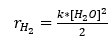# Theoretical reaction rate for water electrolysis

• Erwin123

#### Erwin123

So I'm designing a proces where carbondioxide is converted into methane using the Sabatier reaction. For this reaction hydrogen is required which I'm planning on producing using the electrolysis of water. But I'm having a problem where I need the speed of this reaction to determine the size of the reactor needed. Does anyone know how I can calculate this reaction speed without using experiments? Because the normal equation for reaction rate gives me a value with a reaction rate constant which is obtained experimentally (see the figure on the right for my derived equation for the reaction rate).I hope I can get an answer soon

Last edited:

For an electrolysis, the rate is determined by the current. For the reaction
2H+ + 2e- → H2
dnH2/dt = I/2F
The factor of 2 is because 2 moles of electrons are consumed per mole of hydrogen gas produced.

For an electrolysis, the rate is determined by the current. For the reaction
2H+ + 2e- → H2
dnH2/dt = I/2F
The factor of 2 is because 2 moles of electrons are consumed per mole of hydrogen gas produced.
Thank you one more question what do the I and F stand for? Faraday constant and current of electricity?

Yes. Or current and Faraday constant respectively.